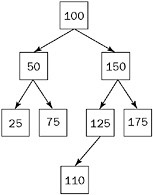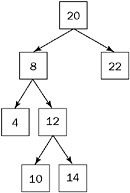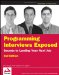# Binary Tree Problems

As previously mentioned, binary trees are the mostly commonly used forms of trees. Here are some typical problems regarding binary trees.

### Preorder Traversal

 Important Informally, a preorder traversal involves walking around the tree in a counterclockwise manner starting at the root, sticking close to the edges, and printing out the nodes as you encounter them. For the tree shown in Figure 5-6, the result is 100, 50, 25, 75, 150, 125, 110, 175. Perform a preorder traversal of a binary search tree, printing the value of each node.Figure 5-6

To discover an algorithm for printing out the nodes in the correct order, you should examine what happens as you print out the nodes. You go to the left as far as possible, come up the tree, go one node to the right, and then go to the left as far as possible, come up the tree again, and so on. The key is to think in terms of subtrees.

The two largest subtrees are rooted at 50 and 150. Note one very important thing about the nodes in these two subtrees: All of the nodes in the subtree rooted at 50 are printed out before any of the nodes in the subtree rooted at 150. In addition, the root node for each subtree is printed out before the rest of the subtree. Generally, for any node in a preorder traversal, you would print the node itself, followed by the left subtree, and then the right subtree. If you begin the printing process at the root node, you would have a recursive definition as follows:

1. Print out the root (or subtree’s root) value.

2. Do a preorder traversal on the left subtree.

3. Do a preorder traversal on the right subtree.

4. Define a Node class similar to the one we created for binary search trees and add a printValue method to the class to print out the node’s value. A preorder traversal is easily coded using recursion:

` void preorderTraversal( Node root ){     if( root == null ) return;     root.printValue();     preorderTraversal( root.getLeft() );     preorderTraversal( root.getRight() ); }`

What’s the running time on this algorithm? Every node is examined once, so it’s O(n).

Note that inorder and postorder traversals are almost identical; all you vary is the order in which the node and subtrees are visited:

` void inorderTraversal( Node root ){     if( root == null ) return;     inorderTraversal( root.getLeft() );     root.printValue();     inorderTraversal( root.getRight() ); } void postorderTraversal( Node root ){     if( root == null ) return;     postorderTraversal( root.getLeft() );     postorderTraversal( root.getRight() );     root.printValue(); }`

The running time is always O(n) for these traversals.

### Preorder Traversal, No Recursion

 Important Perform a preorder traversal of a binary search tree, printing the value of each node, but this time you may not use recursion.

Sometimes recursive algorithms can be replaced with iterative algorithms that accomplish the same task in a fundamentally different manner using different data structures. Consider the data structures you know and think about how they could be helpful. For example, you might try using a list, an array, or another binary tree.

Unfortunately, because recursion is so intrinsic to the definition of a preorder traversal, you may have trouble finding an entirely different iterative algorithm to use in place of the recursive algorithm. In such a case, the best course of action is to understand what is happening in the recursion and try to emulate the process iteratively.

Recursion implicitly uses a stack data structure by placing data on the call stack. That means there should be an equivalent solution that avoids recursion by explicitly using a stack. Assume you have a stack class that can store nodes (writing the stack is a separate problem). Here’s a skeleton class definition for the stack, ignoring all the error handling and cleanup that would normally be required:

` public class NodeStack {     public void push( Node n ){ .... }     public Node pop() { .... } }`

 Tip If you’re not sure what each of these methods does, revisit the stack implementation in Chapter 4. Although that stack was implemented using C/C++, the concepts are identical.

Reexamine your recursive solution to see exactly what is occurring. If you understand how the recursive implementation implicitly stored data on the stack, you can write an iterative implementation that explicitly stores its data on a stack in a similar fashion.

Let’s start with the recursive algorithm:

` Print out the root (or subtree's root) value. Do a preorder traversal on the left subtree. Do a preorder traversal on the right subtree.`

When you first enter the procedure, you print the root node’s value. Next, you recursively call the procedure to traverse the left subtree. When you make this recursive call, the calling procedure’s state is saved on the stack. When the recursive call returns, the calling procedure can pick up where it left off.

In this algorithm, the calling procedure picks up where it left off by doing a traversal of the right subtree. Effectively, the recursive call serves to implicitly store the address of the right subtree on the stack so it can be traversed after the left subtree traversal is complete. Each time you print a node and move to its left child, the right child is first stored on an implicit stack. Whenever there is no child, you return from a recursive call, effectively popping a right child node off the implicit stack so you can continue traversing.

To summarize, the algorithm prints the value of the current node, pushes the right child onto an implicit stack, and moves to the left child. The algorithm pops the stack to obtain a new current node when there are no more children (when it reaches a leaf). This continues until the entire tree has been traversed and the stack is empty.

Before implementing this algorithm, first remove any unnecessary special cases that would make the algorithm more difficult to implement. Instead of coding separate cases for the left and right children,

why not push pointers to both nodes onto the stack? Then all that matters is the order in which the nodes are pushed onto the stack: You need to find an order that enables you to push both nodes onto the stack so that the left node is always popped before the right node.

Because a stack is a last-in-first-out data structure, push the right node onto the stack first, followed by the left node. Instead of examining the left child explicitly, simply pop the first node from the stack, print its value, and push both of its children onto the stack in the correct order. If you start the procedure by pushing the root node onto the stack and then pop, print, and push as described, you can emulate the recursive preorder traversal. To summarize:

` Create the stack Push the root node on the stack While the stack is not empty     Pop a node     If the node is not null         Print its value         Push the node's right child on the stack         Push the node's left child on the stack`

The code (with no error checking) for this algorithm is as follows:

` void preorderTraversal( Node root ){     NodeStack stack = new NodeStack();     stack.push( root );     while( true ){         Node curr = stack.pop();         if( curr == null ) break;         curr.printValue();         Node n = curr.getRight();         if( n != null ) stack.push( n );         n = curr.getLeft();         if( n != null ) stack.push( n );     } }`

What’s the running time for this algorithm? Each node is examined only once and pushed on the stack only once. Therefore, this is still an O(n) algorithm. You don’t have the overhead of many function calls in this implementation. On the other hand, the stack used in this implementation will probably require dynamic memory allocation, so it’s unclear whether the iterative implementation would be more or less efficient than the recursive solution. The point of the problem, however, is to see how well you understand recursion.

### Lowest Common Ancestor

 Important Given the value of two nodes in a binary search tree, find the lowest (nearest) common ancestor. You may assume that both values already exist in the tree. Using the tree shown in Figure 5-7, assume 4 and 14 are the two nodes in question. The lowest common ancestor would be 8 because it’s an ancestor to both 4 and 14 and there is no node lower on the tree that is an ancestor to both 4 and 14.Figure 5-7

Figure 5-7 suggests an intuitive algorithm: Follow the lines up from each of the nodes until they converge. To implement this algorithm, make lists of all the ancestors of both nodes and then search through these two lists to find the first node where they differ. The node right above this divergence will be the lowest common ancestor. This is a good solution, but there is a more efficient one.

The first algorithm doesn’t use any of the special properties of a binary search tree. The method works for any type of tree. Try to use some of the special properties of a binary search tree to help you find the lowest common ancestor more efficiently.

Binary search trees have two special properties. First, every node has zero, one, or two children. This fact doesn’t seem to help find a new algorithm. Second, the left child’s value is less than or equal to the value of the current node, and the right child’s value is greater than or equal to the value of the current node. This property looks more promising.

Looking at the example tree, the lowest common ancestor to 4 and 14, the node with value 8, is different from the other ancestors to 4 and 14 in an important way. All the other ancestors are either greater than both 4 and 14 or less than both 4 and 14. Only 8 is between 4 and 14. You can use this insight to design a better algorithm.

The root node is an ancestor to all nodes because there is a path from it to all other nodes. Therefore, you can start at the root node and follow a path through the common ancestors of both nodes. When your target values are both less than the current node, you go left. When they are both greater, you go right. The first node you encounter that is between your target values is the lowest common ancestor.

Based on this description, and referring to the values of the two nodes as value1 and value2, you can derive the following algorithm:

` Examine the current node If value1 and value2 are both less than the current node's value     Examine the left child If value1 and value2 are both greater than the current node's value     Examine the right child Otherwise     The current node is the lowest common ancestor`

This solution may seem to suggest using recursion because it is a tree and the algorithm has a recursive structure to it, but recursion is not necessary here. Recursion is most useful when moving through multiple branches of a tree or examining some special pattern of nodes. Here you are only traveling down the tree. It’s easy to implement this kind of traversal iteratively:

` Node findLowestCommonAncestor( Node root, int value1,                                int value2 ){     while( root != null ){         int value = root.getValue();         if( value > value1 && value > value2 ){             root = root.getLeft();         } else if( value < value1 && value < value2 ){             root = root.getRight();         } else {             return root;         }    }    return null; // only if empty tree } // Overload it to handle nodes as well Node findLowestCommonAncestor( Node root, Node child1,                                Node child2 ){     if( root == null || child1 == null || child2 == null ){         return null;     }     return findLowestCommonAncestor( root, child1.getValue(),                                      child2.getValue() ); }`

What’s the running time of this algorithm? You are traveling down a path to the lowest common ancestor. Recall that traveling a path to any one node takes O(log(n)). Therefore, this is an O(log(n)) algorithm. In addition, this is slightly more efficient than a similar recursive solution because you don’t have the overhead of repeated function calls.Programming Interviews Exposed: Secrets to Landing Your Next Job, 2nd Edition (Programmer to Programmer)
ISBN: 047012167X
EAN: 2147483647
Year: 2007
Pages: 94

Similar book on Amazon

flylib.com © 2008-2017.
If you may any questions please contact us: flylib@qtcs.net